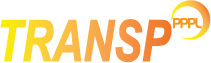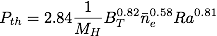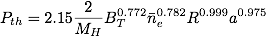# L-H transition

The L-H and H-L transition time can be either set from the namelist, or calculated from power threshold.
By default, TRANSP calculates the L-H power threshold and the pedestal parameters using default settings. To disable these calculations when no needed (e.g. when running TRANSP in analysis mode), the following flag should be set in the namelist:
`NLHMODE=.F.`

### User-defined L-H transition time

NMODEL_L2H_TRANS = 0
To impose the L-H transition time from the namelist
This option also needs the time for the L-H and H-L transition:
TIME_L2H
time (s) of transition from L-mode to H-mode
TIME_H2L
time (s) of transition from H-mode to L-mode

### Model based L-H transition time

TRANSP assumes that the plasma is in H-mode when the power across the separatrix exceeds the power threshold for the L-H transition.

NMODEL_L2H_TRANS = 1
To calculate the L-H transition time from power threshold.

There are two options for the calculation of the L-H power threshold, which can be selected with LPED(1)

LPED(1) = 1
Shimomura scaling [Plasma Phys. Control. Fusion 43 (2001) A385-A394]LPED(1) = 2
Martin scaling [Journal of Physics, Conf. Series 123 (2008) 012033].The input variable CPED(8) can be used to adjust the power threshold, so the plasma is in H-mode if Ploss > CPED(8) x PL-H